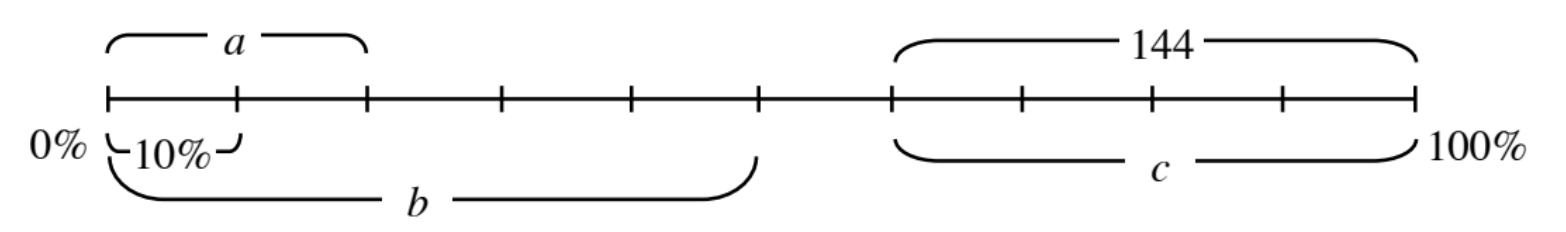### Home > ACC6 > Chapter 5 Unit 12 > Lesson CC2: 5.1.1 > Problem5-9

5-9.

Find the missing values on the diagram below. Assume that each line is evenly divided.If the line is evenly divided, then each interval represents $10\%$ of the total value.
How many intervals are contained within $b$?

There are 5 intervals which means that $b$ represents $50\%$.

Follow the same method for $c$.

$144$ represents $40\%$ of the total value. So how much does $20\%$ represent?